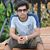# B.TECH 5th sem Digital System Design paper 2017

UniversityGGSIPU, New Delhi
CourseB.TECH
Semester5
SubjectDigital System Design
Year2017
Downloads21
Uploaded By###### Sourabh Bajaj
200

 Question Marks 0 requests (a) What is Package declaration and Package body? Explain it with example. This question has 0 answers so far. 4 0 requests b) Write the capabilities of VHDL. This question has 0 answers so far. 3 0 requests c) Give comparison between PROM, PLA and PAL. This question has 0 answers so far. 3 0 requests d) What is difference between synchronous sequential circuit and Asynchronous sequential circuit?   This question has 0 answers so far. 4 0 requests e) What is operator overloading concept? Explain with an example. This question has 0 answers so far. 4 0 requests f) What is difference between inertial and transport delay? Given examples of each. This question has 0 answers so far. 3 0 requests f) What is difference between inertial and transport delay? Given examples of each. This question has 0 answers so far. 3 0 requests g) Discuss the difference between functions and procedures. Explain with suitable example. This question has 0 answers so far. 4 0 requests a) What What are the different data types in VHDL? Explain all types of data used in VHDL.  This question has 0 answers so far. 8.5 0 requests b) What is generic statement in VHDL? Discuss with examples . This question has 0 answers so far. 4 0 requests a) Write a short note on structural style of modeling.  This question has 0 answers so far. 4.5 0 requests b) Explain with example i) Process statement ii) Case statement This question has 0 answers so far. 8 0 requests a) Write a VHDL code for 4:1 Multiplexer. This question has 0 answers so far. 6 0 requests b) Write VHDL code for 3 to 8 decoder, This question has 0 answers so far. 6.5 0 requests a) Write the VHDL description of the priority encoder. This question has 0 answers so far. 6 0 requests b) Write VHDL code for BCD to seven segment decoder.  This question has 0 answers so far. 6.5 0 requests a) Describe the representation of synchronous sequential machine. Explain the Moore Model in detail.  This question has 0 answers so far. 8 0 requests b) Write the state table, state diagram and VHDL code for JK flip flop.  This question has 0 answers so far. 4.5 0 requests a) Write a VHDL code for state machine to BCD to excess-3 codes Converter. This question has 0 answers so far. 6 0 requests b). Write the VHDL code for 4 bit PIPO shift register. This question has 0 answers so far. 6.5 0 requests a) Explain the Hazards in combinational circuits and sequential circuits. This question has 0 answers so far. 6 0 requests b) Discuss and explain the various clock skew and timing consideration in clocked digital circuits. This question has 0 answers so far. 6.5 0 requests Explain the following:a) Architecture of CPLD This question has 0 answers so far. 6 0 requests Explain the following:b) PLA and PAL architecture This question has 0 answers so far. 6.5
Chat with us